MathScore EduFighter is one of the best math games on the Internet today. You can start playing for free!

## Proportions 2 - Sample Math Practice Problems

The math problems below can be generated by MathScore.com, a math practice program for schools and individual families. References to complexity and mode refer to the overall difficulty of the problems as they appear in the main program. In the main program, all problems are automatically graded and the difficulty adapts dynamically based on performance. Answers to these sample questions appear at the bottom of the page. This page does not grade your responses.

See some of our other supported math practice problems.

### Complexity=5, Mode=triangle

Find the value of 'n'.

 1.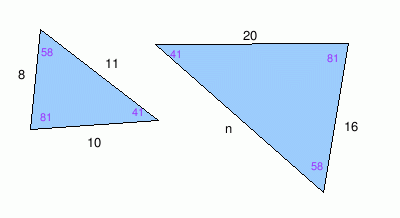n = 2.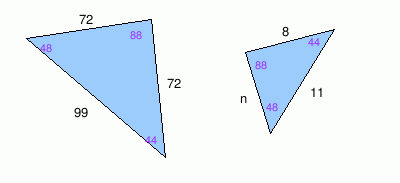n =

### Complexity=8, Mode=parallel

Find the value of 'n'.

 1.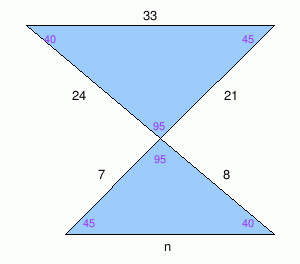n = 2.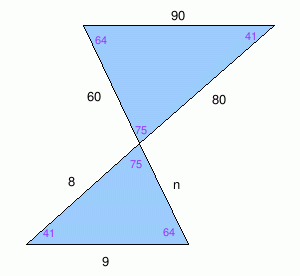n =

### Complexity=10, Mode=trapezoid

Find the value of 'n'.

 1.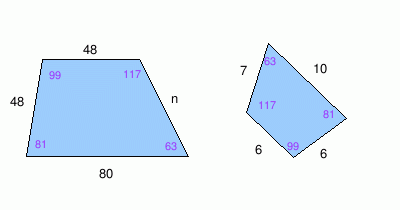n = 2.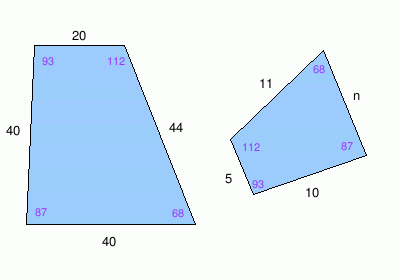n =

### Complexity=20

Find the value of 'n'.

 1.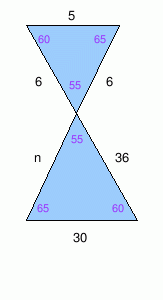n = 2.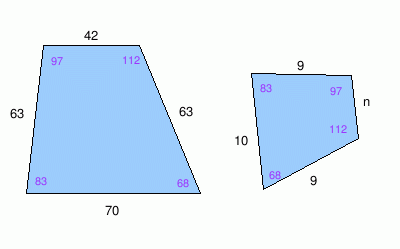n =

### Complexity=5, Mode=triangle

Find the value of 'n'.

1n =
Solution
The two triangles are similar because all their angles are the same but their side lengths are different. This means their corresponding sides are proportional.

Use corresponding sides to create a proportion.

 11n = 1020 = 816

Solve the proportion for n.
11 × 16 = 8 × n
n = 22

2n =
Solution
The two triangles are similar because all their angles are the same but their side lengths are different. This means their corresponding sides are proportional.

Use corresponding sides to create a proportion.

 872 = 1199 = n72

Solve the proportion for n.
n × 99 = 11 × 72
n = 8

### Complexity=8, Mode=parallel

Find the value of 'n'.

1n =
Solution
The two triangles are similar because all their angles are the same but their side lengths are different. This means their corresponding sides are proportional.

Use corresponding sides to create a proportion.

 n33 = 721 = 824

Solve the proportion for n.
n × 24 = 8 × 33
n = 11

2n =
Solution
The two triangles are similar because all their angles are the same but their side lengths are different. This means their corresponding sides are proportional.

Use corresponding sides to create a proportion.

 990 = 880 = n60

Solve the proportion for n.
n × 80 = 8 × 60
n = 6

### Complexity=10, Mode=trapezoid

Find the value of 'n'.

1n =
Solution
The two trapezoids are similar because all their angles are the same but their side lengths are different. This means their corresponding sides are proportional.

Use corresponding sides to create a proportion.

 648 = 648 = 7n = 1080

Solve the proportion for n.
7 × 48 = 6 × n
n = 56

2n =
Solution
The two trapezoids are similar because all their angles are the same but their side lengths are different. This means their corresponding sides are proportional.

Use corresponding sides to create a proportion.

 1040 = 520 = 1144 = n40

Solve the proportion for n.
n × 20 = 5 × 40
n = 10

### Complexity=20

Find the value of 'n'.

1n =
Solution
The two triangles are similar because all their angles are the same but their side lengths are different. This means their corresponding sides are proportional.

Use corresponding sides to create a proportion.

 530 = 6n = 636

Solve the proportion for n.
6 × 30 = 5 × n
n = 36

2n =
Solution
The two triangles are similar because all their angles are the same but their side lengths are different. This means their corresponding sides are proportional.

Use corresponding sides to create a proportion.

 963 = n42 = 963 = 1070

Solve the proportion for n.
n × 70 = 10 × 42
n = 6